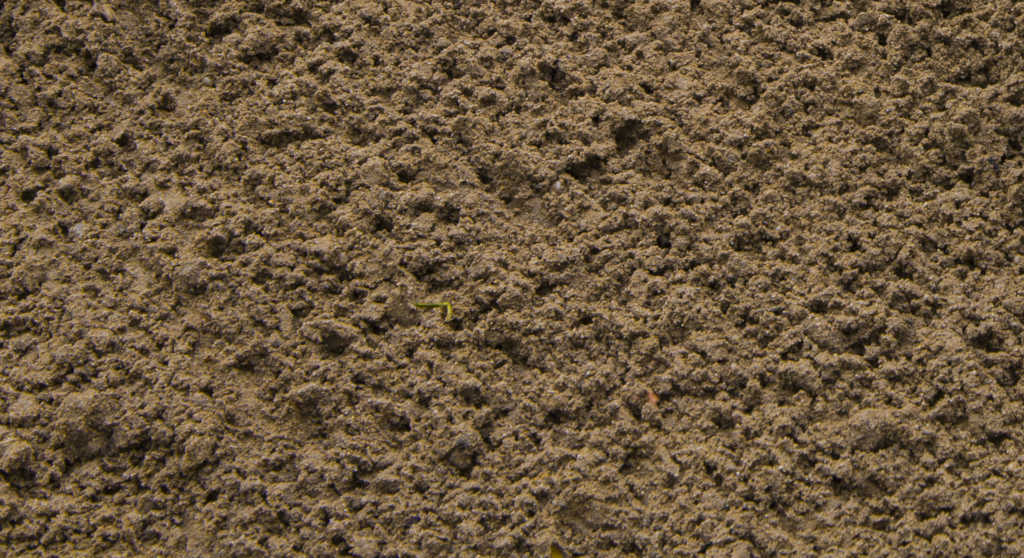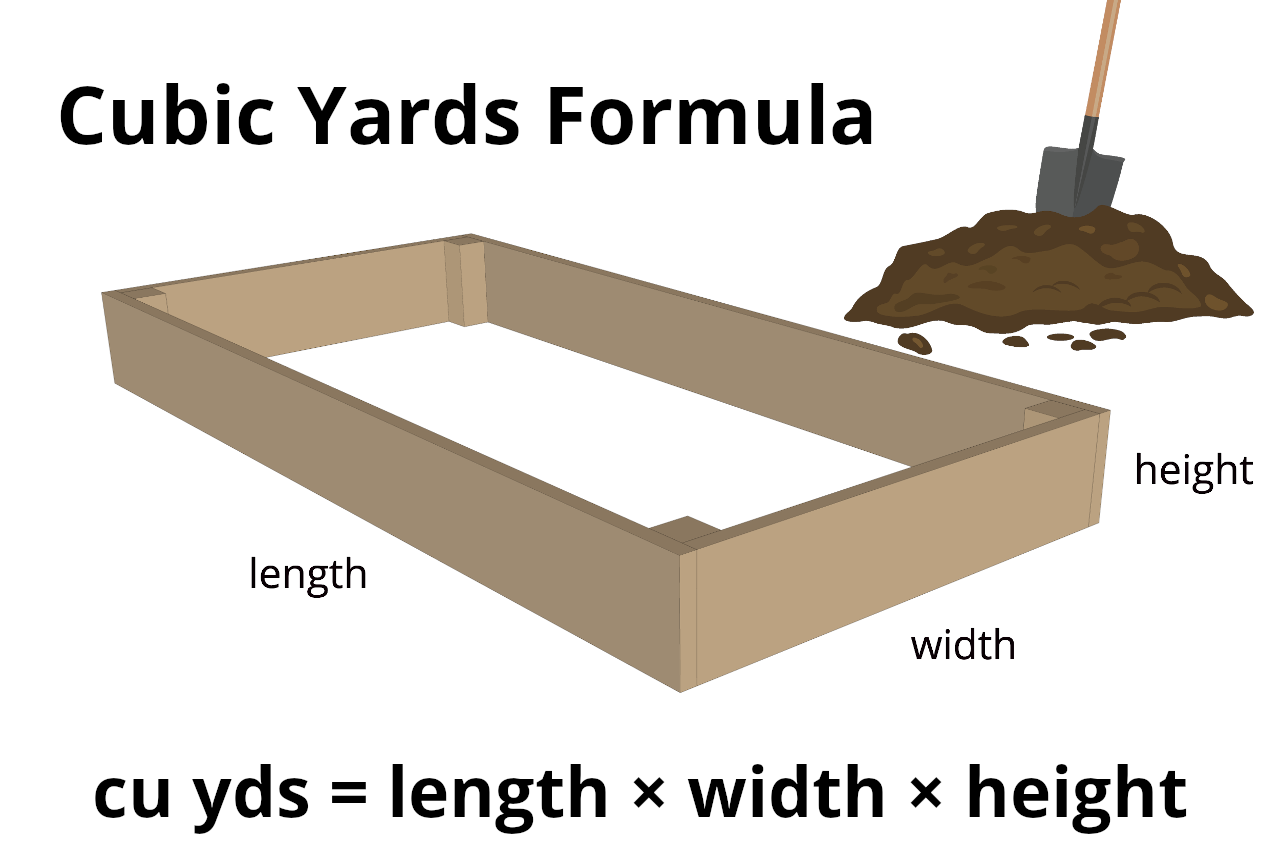# Soil Calculator – Estimate How Much Topsoil You Need

Calculate the soil, topsoil, or fill dirt needed by entering the length, width, and depth of the space you want to fill. Read below for more details.

Find area using our square footage calculator

## How Much Soil You Need:

Cubic yards

Cubic feet
Number of Soil Bags

0.75 cu. ft. bags
Material Weight (dry, loose or packed)

tons
Material Weight (wet)

tons
Learn how we calculated this below

## How Much Topsoil Do You Need?

When you are creating or refreshing a garden or other landscape feature, often you want to add new topsoil to the area. And, in the case of new raised beds, you might need enough new soil to fill your new box completely.

New soil gives your plants the nutrients, air circulation, and water drainage that they require to thrive.To achieve that balance, it’s a good idea to mix soil, compost, and potting blends for the best plant growth. Generally, a good proportion is 60% topsoil, 30% compost, and 10% potting mix.

You may also need fill dirt for landscaping projects and hardscapes, which is used to fill in large volumes.

### Steps to Estimate Soil Volume

Adding soil can be a substantial investment in time and money. To minimize work and expense, follow these instructions for getting the right amount of soil that you need.

1.   Using a tape measure, measure the length and width of the area(s) you want to fill. It’s best to write these numbers down.

2.   If the space is complex, break the area into smaller, regularly shaped sections, like in the diagram below. Then measure each section separately.

3.   Decide on the depth of the new soil that you want. For refreshing gardens, usually, 3-6 inches (76-152 mm) of new soil is sufficient. If you have a hard border or a raised bed, measure the depth of the partition. Remember that soil will settle, so be a little generous with this measurement.

4.   By far, the easiest way to calculate quantities is to use the soil calculator above! Enter your measurements, hit calculate, and it’s all worked out for you: cubic feet, yards, even the number of bags. And metric too.

Sometimes topsoil and compost are sold by weight, which varies depending on what the material is. In the US, a 40-pound bag measures between 0.5 and 0.75 cubic feet. The Soil Calculator bag estimator is based on 0.75 cubic feet per bag.

### Calculations

If you want to do the calculations by hand, then skip Step 4 above, and do the following:

1.   Make sure all measurements are in the same units (usually feet or meters).

2.   Then multiply them all together to find the volume in cubic feet (length × width × depth).

3.   Add together the separate volumes if you divided your space into sections.

4.   The result is the volume of soil required for your project. You will need to figure the number of bags depending on what size you buy.No matter how you get your total, you should consider buying in bulk by the cubic yard (or cubic meter) if you need a lot of material. The calculator above provides this volume for you.

For hand calculators, divide your total cubic feet by 27 (3×3×3) to get cubic yards.

Be sure to check your calculations and confirm your measurements using a cubic yards calculator. Give this number to your supplier, who will usually deliver the soil to your location for a small fee.

Soon your plants will be enjoying their new environment, and you will be enjoying your happy plants!

Check out our mulch calculator to estimate mulch for your garden bed, or see our gravel calculator to estimate stone, gravel, and other landscaping materials.

## How Much is a Yard of Dirt?

At this point, you still might be wondering just how much is a yard of dirt?

One cubic yard is equal to a space that is one yd wide, one yd long, and one yd deep. The table below shows the size of the space equal to one cubic yard of soil in other units.

1 cu yd = 1 yd x 1 yd x 1 yd 3 ft x 3 ft x 3 ft 36 in x 36 in x 36 in 0.9144 m x 0.9144 m x 0.9144 m 91.44 cm x 91.44 cm x 91.44 cm

## How to Estimate Soil in Tons

You can calculate how much soil you need in tons by multiplying its density by the volume.

### How Much Does a Yard of Topsoil Weigh?

So, how much does a cubic yard of dirt weigh? One yard of dry or loose soil weighs 1.0 – 1.3 tons, while one yard of wet or compacted soil weighs 1.5 – 1.7 tons.

### Calculate the Total Weight

Once you know the density and total volume, use the following formula to calculate how much soil you need in tons.

weight (tons) = volume (yds) × density (tons/yd)

Thus, the amount of soil needed in tons is equal to the volume in yards times the density.

You might also be interested in out stone and sand calculators for more landscaping material estimates.